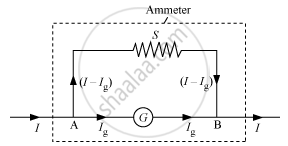PUC Karnataka Science Class 12Department of Pre-University Education, Karnataka
Share

# Can a galvanometer as such be used for measuring the current? Explain. - PUC Karnataka Science Class 12 - Physics

#### Question

Can a galvanometer as such be used for measuring the current? Explain.

#### Solution

Yes a galvanometer can be used for measuring the current

Conversion of a galvanometer into an ammeterA shunt (low resistance) is connected in parallel with a  galvanometer.

S=(I_g/(I-I_g))G

Here,

I = total current in circuit

G = resistance of the galvanometer

S = resistance of the shunt

Ig = current through the galvanometer

Is there an error in this question or solution?

#### Video TutorialsVIEW ALL 

Solution Can a galvanometer as such be used for measuring the current? Explain. Concept: Moving Coil Galvanometer.
S